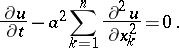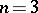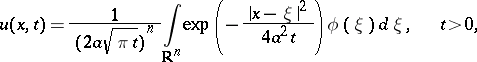# Thermal-conductance equation

(diff) ← Older revision | Latest revision (diff) | Newer revision → (diff)

heat equation

The homogeneous partial differential equationThis equation is the simplest example of a parabolic partial differential equation. Forit describes the process of heat diffusion in a solid body. The first boundary value problem (in a cylindrical domain) and the Cauchy–Dirichlet problem (in a half-space) are the fundamental well-posed problems for the thermal-conductance equation. A solution to the characteristic (Cauchy) problem can be given in explicit form:whereis a fixed continuous uniformly bounded function on.

How to Cite This Entry:
Thermal-conductance equation. Encyclopedia of Mathematics. URL: http://encyclopediaofmath.org/index.php?title=Thermal-conductance_equation&oldid=17398
This article was adapted from an original article by A.P. Soldatov (originator), which appeared in Encyclopedia of Mathematics - ISBN 1402006098. See original article